Sep. 18, 2016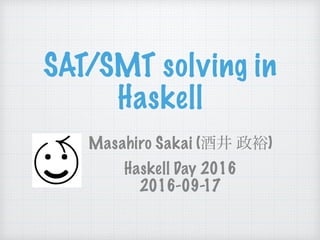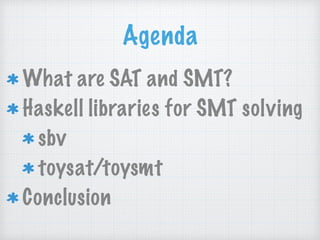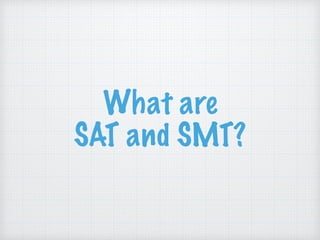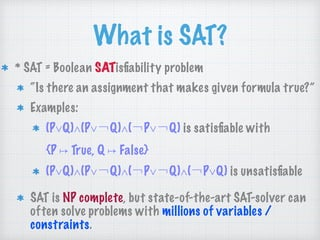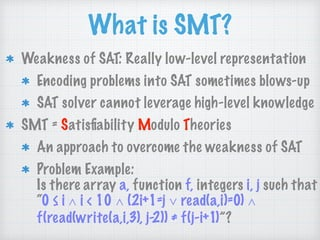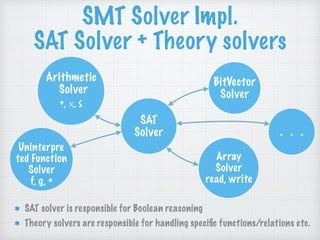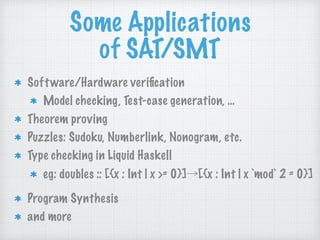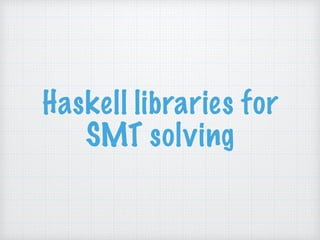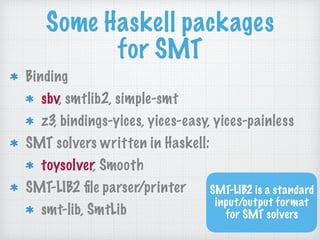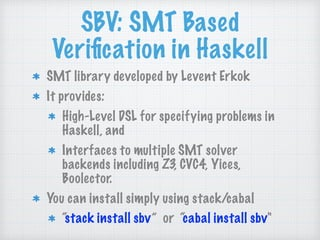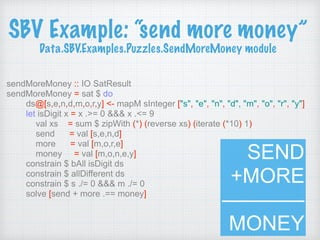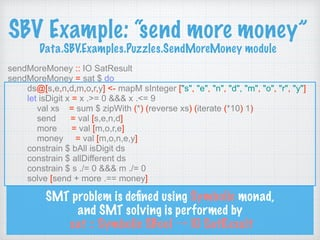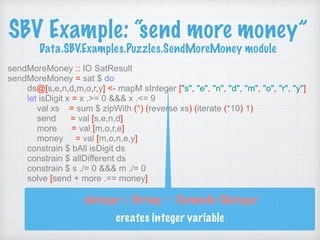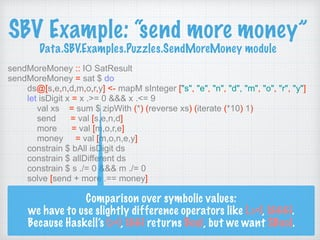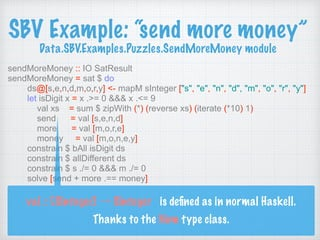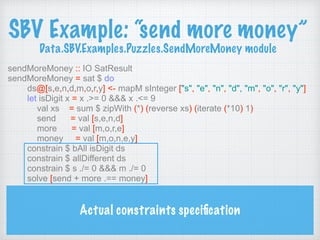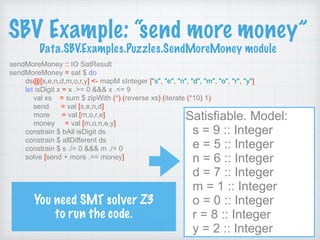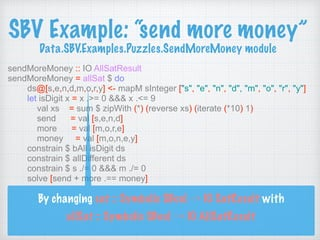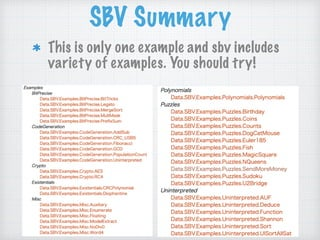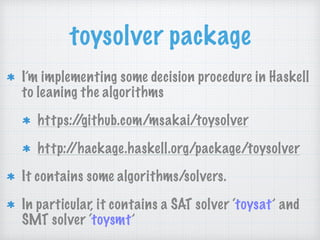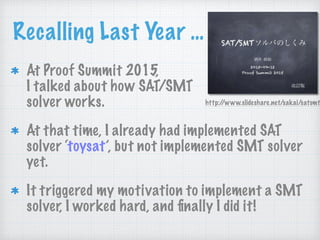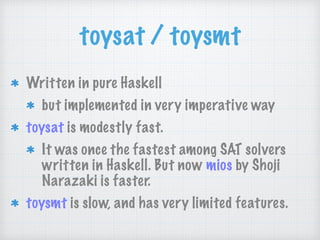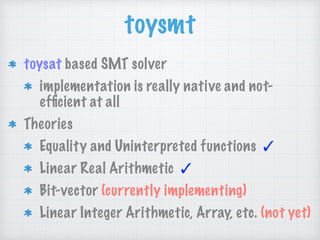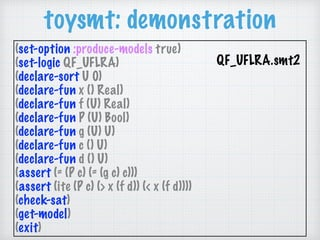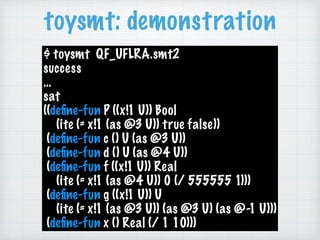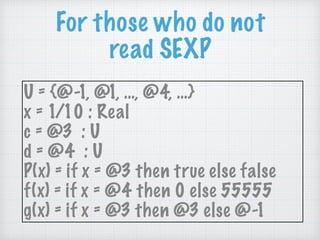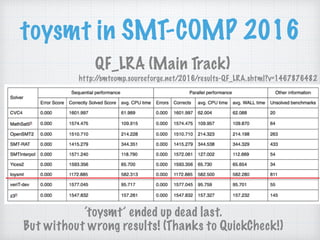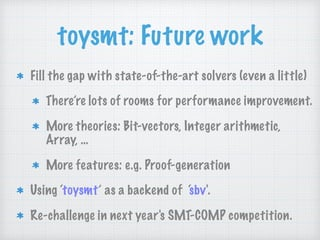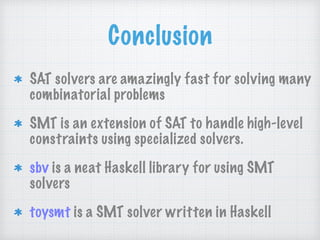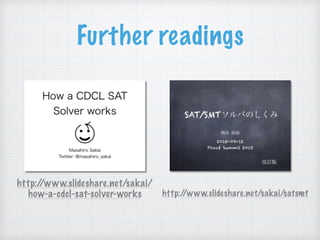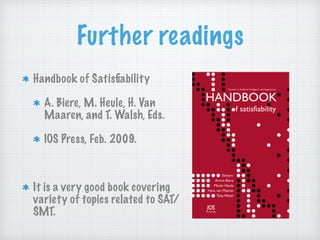1 of 32

• 1. SAT/SMT solving in  Haskell Masahiro Sakai (酒井 政裕)  Haskell Day 2016 2016-09-17
• 2. Self Introduction  Masahiro Sakai Twitter: @masahiro_sakai github: https://github.com/msakai/ G+: https://plus.google.com/+MasahiroSakai Translated “Software Abstractions” and TaPL into Japanese with colleagues Interests: Categorical Programming, Theorem Proving / Decision Procedures,  …
• 3. Agenda What are SAT and SMT? Haskell libraries for SMT solving sbv toysat/toysmt Conclusion
• 4. What are  SAT and SMT?
• 5. What is SAT? * SAT = Boolean SATisﬁability problem “Is there an assignment that makes given formula true?” Examples: (P∨Q)∧(P∨￢Q)∧(￢P∨￢Q) is satisﬁable with  {P ↦ True, Q ↦ False} (P∨Q)∧(P∨￢Q)∧(￢P∨￢Q)∧(￢P∨Q) is unsatisﬁable SAT is NP complete, but state-of-the-art SAT-solver can often solve problems with millions of variables / constraints.
• 6. What is SMT? Weakness of SAT: Really low-level representation Encoding problems into SAT sometimes blows-up SAT solver cannot leverage high-level knowledge SMT = Satisﬁability Modulo Theories An approach to overcome the weakness of SAT Problem Example:  Is there array a, function f, integers i, j such that  “0 ≤ i ∧ i < 10 ∧ (2i+1=j ∨ read(a,i)=0) ∧ f(read(write(a,i,3), j-2)) ≠ f(j-i+1)”?
• 7. SMT Solver Impl.  SAT Solver + Theory solvers SAT solver is responsible for Boolean reasoning Theory solvers are responsible for handling speciﬁc functions/relations etc. SAT Solver Arithmetic Solver  +, ×, ≤ BitVector Solver Uninterpre ted Function  Solver  f, g, = Array  Solver  read, write   ・・・
• 8. Some Applications  of SAT/SMT Software/Hardware veriﬁcation Model checking, Test-case generation, … Theorem proving Puzzles: Sudoku, Numberlink, Nonogram, etc. Type checking in Liquid Haskell eg: doubles :: [{x : Int | x >= 0}]→[{x : Int | x `mod` 2 = 0}] Program Synthesis and more
• 9. Haskell libraries for SMT solving
• 10. Some Haskell packages for SMT Binding sbv, smtlib2, simple-smt z3, bindings-yices, yices-easy, yices-painless SMT solvers written in Haskell: toysolver, Smooth SMT-LIB2 ﬁle parser/printer smt-lib, SmtLib SMT-LIB2 is a standard  input/output format for SMT solvers
• 11. SBV: SMT Based Veriﬁcation in Haskell SMT library developed by Levent Erkok It provides: High-Level DSL for specifying problems in Haskell, and Interfaces to multiple SMT solver backends including Z3, CVC4, Yices, Boolector. You can install simply using stack/cabal “stack install sbv” or “cabal install sbv"
• 12. SBV Example: “send more money”  Data.SBV.Examples.Puzzles.SendMoreMoney module sendMoreMoney :: IO SatResult sendMoreMoney = sat \$ do ds@[s,e,n,d,m,o,r,y] <- mapM sInteger ["s", "e", "n", "d", "m", "o", "r", "y"] let isDigit x = x .>= 0 &&& x .<= 9 val xs = sum \$ zipWith (*) (reverse xs) (iterate (*10) 1) send = val [s,e,n,d] more = val [m,o,r,e] money = val [m,o,n,e,y] constrain \$ bAll isDigit ds constrain \$ allDifferent ds constrain \$ s ./= 0 &&& m ./= 0 solve [send + more .== money] SEND  +MORE ————  MONEY
• 13. SBV Example: “send more money”  Data.SBV.Examples.Puzzles.SendMoreMoney module sendMoreMoney :: IO SatResult sendMoreMoney = sat \$ do ds@[s,e,n,d,m,o,r,y] <- mapM sInteger ["s", "e", "n", "d", "m", "o", "r", "y"] let isDigit x = x .>= 0 &&& x .<= 9 val xs = sum \$ zipWith (*) (reverse xs) (iterate (*10) 1) send = val [s,e,n,d] more = val [m,o,r,e] money = val [m,o,n,e,y] constrain \$ bAll isDigit ds constrain \$ allDifferent ds constrain \$ s ./= 0 &&& m ./= 0 solve [send + more .== money] SMT problem is deﬁned using Symbolic monad, and SMT solving is performed by  sat :: Symbolic SBool → IO SatResult
• 14. SBV Example: “send more money”  Data.SBV.Examples.Puzzles.SendMoreMoney module sendMoreMoney :: IO SatResult sendMoreMoney = sat \$ do ds@[s,e,n,d,m,o,r,y] <- mapM sInteger ["s", "e", "n", "d", "m", "o", "r", "y"] let isDigit x = x .>= 0 &&& x .<= 9 val xs = sum \$ zipWith (*) (reverse xs) (iterate (*10) 1) send = val [s,e,n,d] more = val [m,o,r,e] money = val [m,o,n,e,y] constrain \$ bAll isDigit ds constrain \$ allDifferent ds constrain \$ s ./= 0 &&& m ./= 0 solve [send + more .== money] sInteger :: String → Symbolic SInteger  creates integer variable
• 15. SBV Example: “send more money”  Data.SBV.Examples.Puzzles.SendMoreMoney module sendMoreMoney :: IO SatResult sendMoreMoney = sat \$ do ds@[s,e,n,d,m,o,r,y] <- mapM sInteger ["s", "e", "n", "d", "m", "o", "r", "y"] let isDigit x = x .>= 0 &&& x .<= 9 val xs = sum \$ zipWith (*) (reverse xs) (iterate (*10) 1) send = val [s,e,n,d] more = val [m,o,r,e] money = val [m,o,n,e,y] constrain \$ bAll isDigit ds constrain \$ allDifferent ds constrain \$ s ./= 0 &&& m ./= 0 solve [send + more .== money] Comparison over symbolic values: we have to use slightly difference operators like (.>=), (&&&). Because Haskell’s (>=), (&&) returns Bool, but we want SBool.
• 16. SBV Example: “send more money”  Data.SBV.Examples.Puzzles.SendMoreMoney module sendMoreMoney :: IO SatResult sendMoreMoney = sat \$ do ds@[s,e,n,d,m,o,r,y] <- mapM sInteger ["s", "e", "n", "d", "m", "o", "r", "y"] let isDigit x = x .>= 0 &&& x .<= 9 val xs = sum \$ zipWith (*) (reverse xs) (iterate (*10) 1) send = val [s,e,n,d] more = val [m,o,r,e] money = val [m,o,n,e,y] constrain \$ bAll isDigit ds constrain \$ allDifferent ds constrain \$ s ./= 0 &&& m ./= 0 solve [send + more .== money] val :: [SInteger] → SInteger is deﬁned as in normal Haskell. Thanks to the Num type class.
• 17. SBV Example: “send more money”  Data.SBV.Examples.Puzzles.SendMoreMoney module sendMoreMoney :: IO SatResult sendMoreMoney = sat \$ do ds@[s,e,n,d,m,o,r,y] <- mapM sInteger ["s", "e", "n", "d", "m", "o", "r", "y"] let isDigit x = x .>= 0 &&& x .<= 9 val xs = sum \$ zipWith (*) (reverse xs) (iterate (*10) 1) send = val [s,e,n,d] more = val [m,o,r,e] money = val [m,o,n,e,y] constrain \$ bAll isDigit ds constrain \$ allDifferent ds constrain \$ s ./= 0 &&& m ./= 0 solve [send + more .== money] Actual constraints speciﬁcation
• 18. SBV Example: “send more money”  Data.SBV.Examples.Puzzles.SendMoreMoney module sendMoreMoney :: IO SatResult sendMoreMoney = sat \$ do ds@[s,e,n,d,m,o,r,y] <- mapM sInteger ["s", "e", "n", "d", "m", "o", "r", "y"] let isDigit x = x .>= 0 &&& x .<= 9 val xs = sum \$ zipWith (*) (reverse xs) (iterate (*10) 1) send = val [s,e,n,d] more = val [m,o,r,e] money = val [m,o,n,e,y] constrain \$ bAll isDigit ds constrain \$ allDifferent ds constrain \$ s ./= 0 &&& m ./= 0 solve [send + more .== money] Satisfiable. Model: s = 9 :: Integer e = 5 :: Integer n = 6 :: Integer d = 7 :: Integer m = 1 :: Integer o = 0 :: Integer r = 8 :: Integer y = 2 :: Integer You need SMT solver Z3  to run the code.
• 19. SBV Example: “send more money”  Data.SBV.Examples.Puzzles.SendMoreMoney module sendMoreMoney :: IO AllSatResult sendMoreMoney = allSat \$ do ds@[s,e,n,d,m,o,r,y] <- mapM sInteger ["s", "e", "n", "d", "m", "o", "r", "y"] let isDigit x = x .>= 0 &&& x .<= 9 val xs = sum \$ zipWith (*) (reverse xs) (iterate (*10) 1) send = val [s,e,n,d] more = val [m,o,r,e] money = val [m,o,n,e,y] constrain \$ bAll isDigit ds constrain \$ allDifferent ds constrain \$ s ./= 0 &&& m ./= 0 solve [send + more .== money] By changing sat :: Symbolic SBool → IO SatResult with  allSat :: Symbolic SBool → IO AllSatResult
• 20. SBV Summary This is only one example and sbv includes variety of examples. You should try!
• 21. toysolver package I’m implementing some decision procedure in Haskell to leaning the algorithms https://github.com/msakai/toysolver http://hackage.haskell.org/package/toysolver It contains some algorithms/solvers. In particular, it contains a SAT solver ‘toysat’ and SMT solver ‘toysmt’
• 22. Recalling Last Year … At Proof Summit 2015,  I talked about how SAT/SMT  solver works. At that time, I already had implemented SAT solver ‘toysat’, but not implemented SMT solver yet. It triggered my motivation to implement a SMT solver, I worked hard, and ﬁnally I did it! http://www.slideshare.net/sakai/satsmt
• 23. toysat / toysmt Written in pure Haskell but implemented in very imperative way toysat is modestly fast. It was once the fastest among SAT solvers written in Haskell. But now mios by Shoji Narazaki is faster. toysmt is slow, and has very limited features.
• 24. toysmt toysat based SMT solver implementation is really native and not- efﬁcient at all Theories Equality and Uninterpreted functions ✓ Linear Real Arithmetic ✓ Bit-vector (currently implementing) Linear Integer Arithmetic, Array, etc. (not yet)
• 25. toysmt: demonstration (set-option :produce-models true) (set-logic QF_UFLRA) (declare-sort U 0) (declare-fun x () Real) (declare-fun f (U) Real) (declare-fun P (U) Bool) (declare-fun g (U) U) (declare-fun c () U) (declare-fun d () U) (assert (= (P c) (= (g c) c))) (assert (ite (P c) (> x (f d)) (< x (f d)))) (check-sat) (get-model) (exit) QF_UFLRA.smt2
• 26. toysmt: demonstration \$ toysmt QF_UFLRA.smt2  success … sat ((deﬁne-fun P ((x!1 U)) Bool  (ite (= x!1 (as @3 U)) true false))  (deﬁne-fun c () U (as @3 U))  (deﬁne-fun d () U (as @4 U))  (deﬁne-fun f ((x!1 U)) Real  (ite (= x!1 (as @4 U)) 0 (/ 555555 1)))  (deﬁne-fun g ((x!1 U)) U  (ite (= x!1 (as @3 U)) (as @3 U) (as @-1 U)))  (deﬁne-fun x () Real (/ 1 10)))
• 27. For those who do not read SEXP U = {@-1, @1, …, @4, …} x = 1/10 : Real c = @3 : U d = @4 : U P(x) = if x = @3 then true else false f(x) = if x = @4 then 0 else 55555 g(x) = if x = @3 then @3 else @-1
• 28. toysmt in SMT-COMP 2016 QF_LRA (Main Track) http://smtcomp.sourceforge.net/2016/results-QF_LRA.shtml?v=1467876482 ‘toysmt’ ended up dead last.  But without wrong results! (Thanks to QuickCheck!)
• 29. toysmt: Future work Fill the gap with state-of-the-art solvers (even a little) There’re lots of rooms for performance improvement. More theories: Bit-vectors, Integer arithmetic, Array, … More features: e.g. Proof-generation Using ‘toysmt’ as a backend of ‘sbv'. Re-challenge in next year's SMT-COMP competition.
• 30. Conclusion SAT solvers are amazingly fast for solving many combinatorial problems SMT is an extension of SAT to handle high-level constraints using specialized solvers. sbv is a neat Haskell library for using SMT solvers toysmt is a SMT solver written in Haskell
• 31. Further readings http://www.slideshare.net/sakai/satsmt http://www.slideshare.net/sakai/ how-a-cdcl-sat-solver-works
• 32. Further readings Handbook of Satisﬁability A. Biere, M. Heule, H. Van Maaren, and T. Walsh, Eds. IOS Press, Feb. 2009. It is a very good book covering variety of topics related to SAT/ SMT.